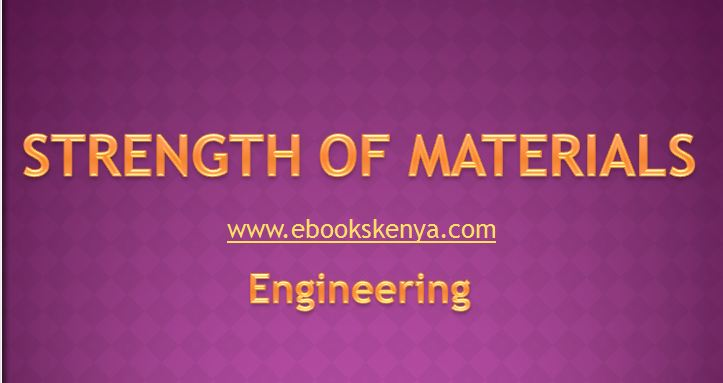# Strength of Materials KNEC notesINTRODUCTION

Strength of materials is a module unit that deals with materials and their application in structural engineering and tool room processes.

### Stress and Strain

• Determination of elastic constants

### Shearing Forces and Bending Moments

• Determination of shearing force and bending moment

### Bending Stresses in Beams

• Verification of pure bending theory
• Cantilevers
• Simply supported beams

### Deflection of Beams

• Determination of Young’s modulus
• Cantilevers
• Simply supported beams
• Verification of the principles of super position of deflection in beams
• Determination of fixing moments for loaded beams

### Thin Cylinders and Shells

• Determination of the circumferential and longitudinal strains and stresses in pressurized thin vessels

### Torsion

• Statement of the simple theory of torsion of circular section bars.
• Explanation of parameters in the simple theory of torsion equation
• Derivation from first principle the relationship between strain and twist per length.
• Using the equation to develop other equations
• Application of the equations to solve problems in torsion
• Strain Energy
• Definition of strain energy
• Derivation of expressions for strain energy from first principles of different loading conditions
• Definition of resilience and proof resistance
• Derivation of an expression for strain energy of a three dimensional principal stress system.
• Definition of modules of resistance
• Definition of a maximum instantaneous stress
• Definition of maximum instantaneous extension
• Derivation of an expression for strain energy due to instantaneous loading
• Statement of Castigliano’s 1st theorem for deflection
• Application of Castigliano theorem to derive expressions for deflection on various beam loading system

### Springs

• Definition of a spring
• Identification of various types of springs
• Derivation of stress expression of springs
• Maximum shear stress for coiled springs
• Maximum bending stress for a close coiled spring sunder axial torque
• Maximum bending stress for semi-elliptic spring under central load
• Proof stress for semi-elliptic spring under central load
• Max. bending stress for quarter-elliptic spring
• Max. bending stress for plain spiral spring
• Derivation of deflection of springs
• Derivation of spring rate expression for various springs
• Application of the expressions to solve spring problems
• Derivation expressions for stiffness of springs
(Visited 2,334 times, 1 visits today)

## 2 thoughts on “Strength of Materials KNEC notes”

1.Douglas says:

Now can I have those topics’ notes

1.Haron says:

Request you to send me the notes